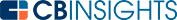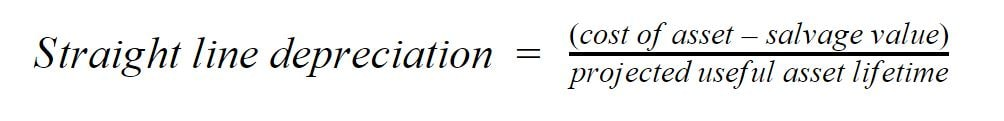# What is the Straight Line Depreciation Formula?Businesses calculate depreciation expenses to determine how to reduce a capital asset’s value over its useful lifetime for tax purposes.

Straight line depreciation is the simplest depreciation method for determining the asset value to write off on the company’s tax return each year. Using this method, the asset’s value is reduced uniformly. This means that the amount of the tax deduction is the same annually until the asset’s value is reduced to its salvage value.

## How to calculate straight line depreciation

To calculate straight line depreciation, follow these steps:

• First, determine the cost of the asset.
• Next, determine the salvage value.
• Then determine the projected useful lifetime of the asset.
• You can now find the straight line depreciation by subtracting the salvage value of the asset from the cost of the asset and then dividing the result by the projected useful lifetime of the asset.

This gives you the annual depreciation of an asset in a straightforward way. Here’s what the formula looks like:Here’s a breakdown of the terms used in the formula:

Cost of asset: This is the price the asset was purchased for.

Salvage value: This is the value of the asset at the end of its projected useful lifetime.

Projected useful asset lifetime: This is the period of time an asset is estimated to be useful for.

Another useful formula is the straight line depreciation rate. This basically states the same information in a different way and is calculated as follows:This tells you the fractional amount of lost value per year.

## Straight line depreciation example

Consider a company called Linear Informatics that buys a device meant to streamline operations for \$15,000. This device has a projected useful lifetime of 5 years and a salvage value of \$1,000. To calculate the straight line depreciation, the accountants at Linear Informatics will use the following values:

Cost of asset: \$15,000

Salvage value of asset: \$1,000

With this information, they’ll calculate the straight line depreciation as follows:We calculated a straight line depreciation amount of \$2,800. This means the device will depreciate by \$2,800 every year. Now, take a look at the table below to see how the value of the device depreciates over its useful lifetime.At the end of the device’s lifetime of 5 years, the device reaches its salvage value of \$1,000 as expected. This is a way of checking to see if the straight line depreciation was calculated correctly.

## Pros and cons of the straight line depreciation formula

The main advantage of the straight line depreciation formula is its simplicity. With just 3 variables, the amount of depreciation can be calculated. However, the projected useful lifetime of the asset is an estimate that can throw off the calculation over time. For example, the value of technology can sometimes deteriorate more rapidly than expected. Events like this can throw off a company’s projections.

The straight line depreciation formula is a simple way of calculating the cost of an asset over time. It’s calculated by subtracting the salvage value of an asset from its cost. This is then divided by the estimated projected useful lifetime of the asset. While it’s simple to calculate straight line depreciation, there are drawbacks, such as possible inaccuracies with the projected estimated useful lifetime of the asset in question.

You may also like# www.cbinsights.com